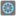• # array index failure but how

## Question related to missionEven the Last

def checkio(array): """ sums even-indexes elements and multiply at the last """ sum = 0 product = 0

```if len(array) == 1:
return array*array

elif len(array) == 0:
return 0

else:
for a in range(0,len(array)-1, 2):
n = array[a]
sum += n
product = sum * array[-1]

return product
```

This one gives Assertion error on ([1, 3, 5]) == 30 even if my result is 30. If I add the following line:

elif len(array) == 3: return (array+array)*array[-1]

to overcome the assertion problem, it is giving me the following failure:

Your result is -4200, but correct result is -1700 for test data (-89,-86,13,-69,94,-75,66,97,-50)

How come is my result different? And also why is my code not working on (assert checkio([1, 3, 5]) == 30, "(1+5)*5=30" even if I find the same result?